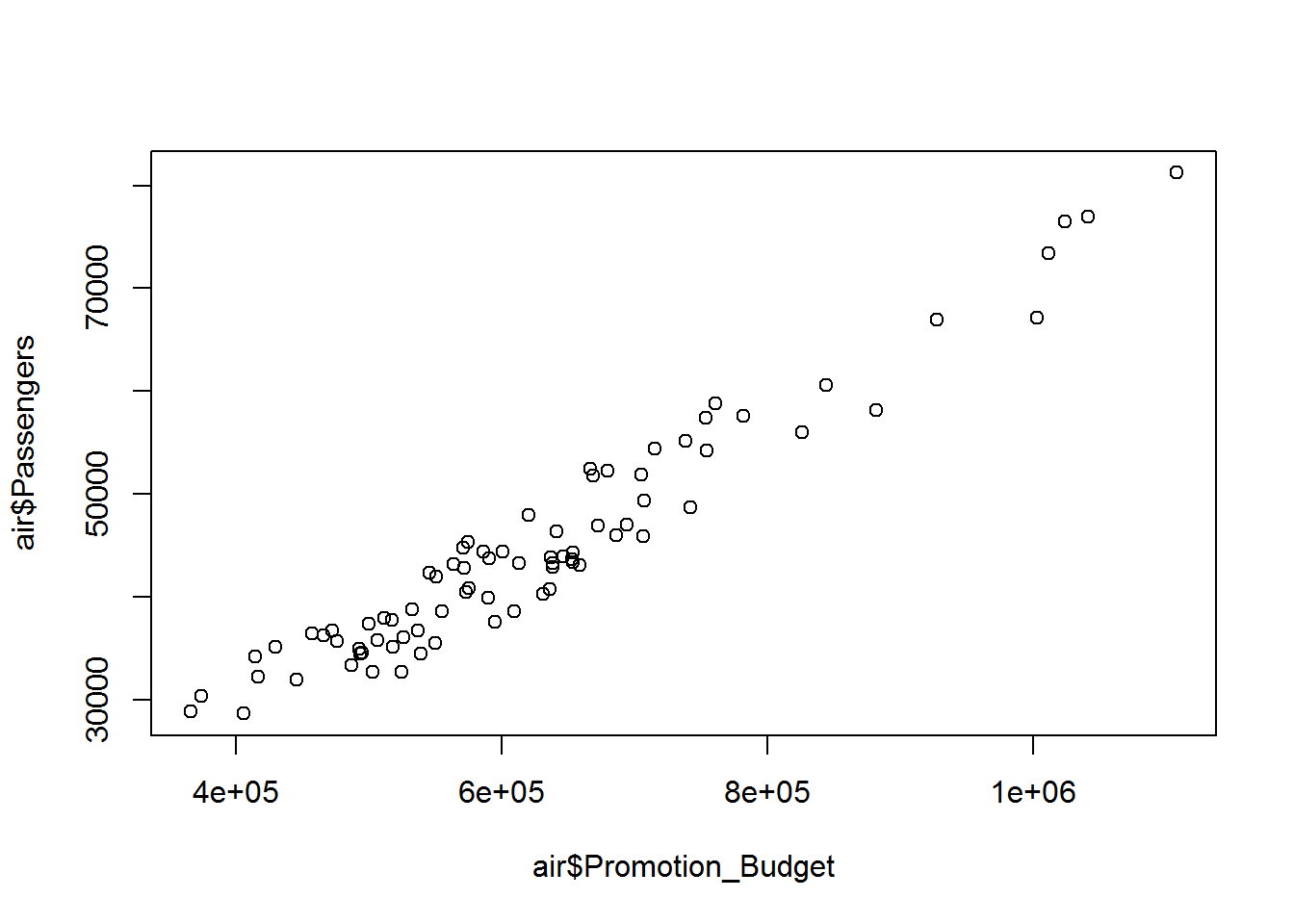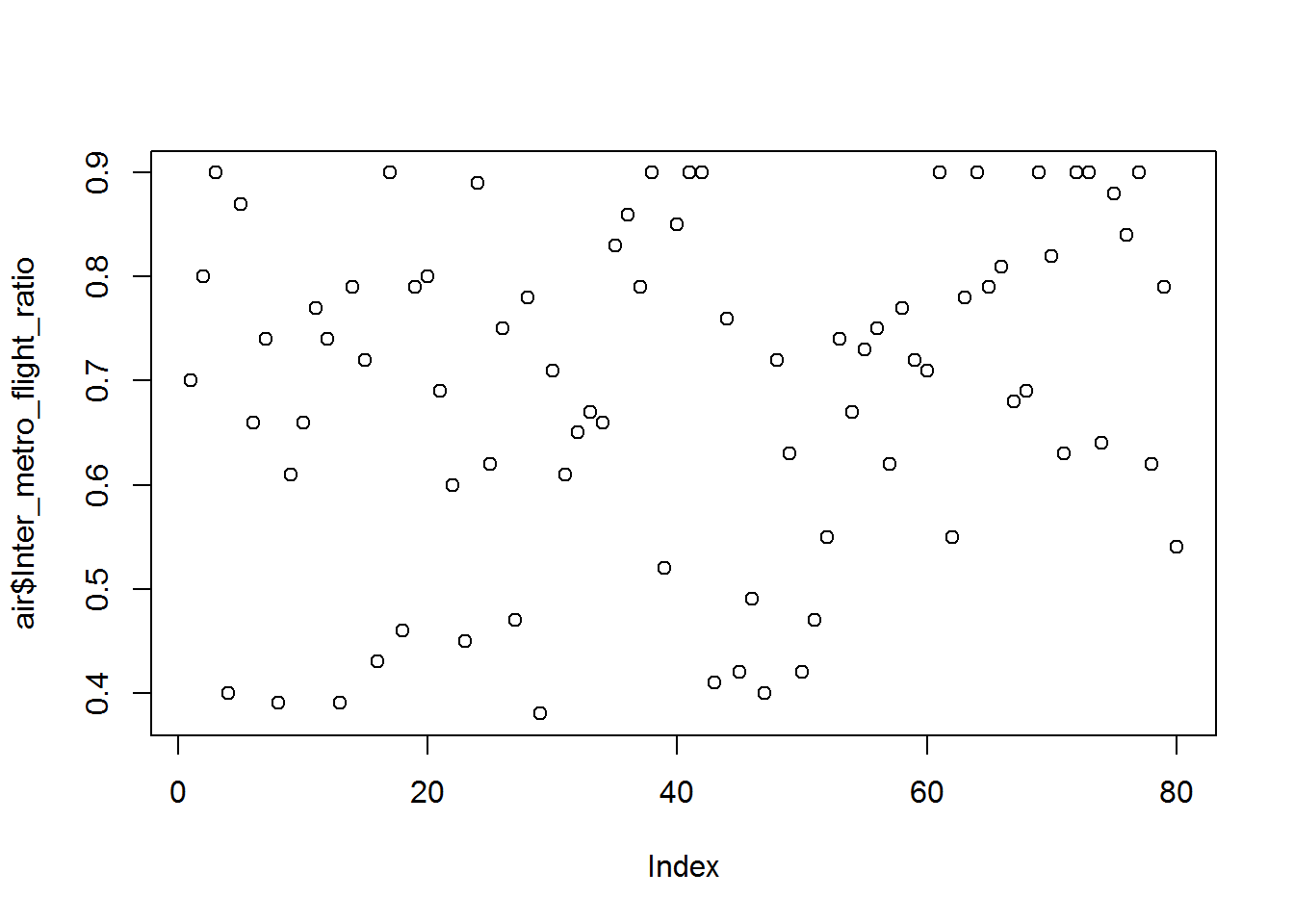• No products in the cart.

# 203.1.5 Practice : Regression Line Fitting in R

In previous section, we studied about  Regression Introduction

#### Practice : Regression Line Fitting

1. Import Dataset: AirPassengers.csv
2. Find the correlation between Promotion_Budget and Passengers
3. Draw a scatter plot between Promotion_Budget and Passengers. Is there any any pattern between Promotion_Budget and Passengers?
4. Build a linear regression model on Promotion_Budget and Passengers.
5. If the Promotion_Budget is 650,000 how many passenger’s can be expected in that week?
6. Build a regression line to predict the passengers using Inter_metro_flight_ratio

#### Solution

1. Import Dataset: AirPassengers/AirPassengers.csv
air <- read.csv ("R dataset\\AirPassengers\\AirPassengers.csv")
1. Find the correlation between Promotion_Budget and Passengers
cor(air$Passengers,air$Promotion_Budget)
##  0.965851

The promotional budget and passengers the correlation is 96% which is a clear indicator of strong relationship.

1. Draw a scatter plot between Promotion_Budget and Passengers. Is there any any pattern between Promotion_Budget and Passengers?
plot(air$Promotion_Budget,air$Passengers)There is a positive pattern between Promotion budget and passengers. We can see there is a very high correlation between these two variables as the promotional budget increase i.e reducing the ticket fares giving the coupons definitely number of passengers are really growing high. If a very less amount is spend on promotional budget in a particular week then the numbers of passengers are low.

1. Build a linear regression model on Promotion_Budget and Passengers.

For building the regression line we have to use a function called a linear model, lm is the abbreviation for linear model, then use the name of variable whose value needed to be predicted that is the number of passenger in this particular problem, use the symbol ‘~’ tilde then promotion budget which is in the dataset of Air passengers. We need to observe the code, where lm is abbreviation of linear model, Y is the number of passengers and X is the promotional budget.

model1<-lm(air$Passengers~air$Promotion_Budget,data=air)
summary(model1)
##
## Call:
## lm(formula = air$Passengers ~ air$Promotion_Budget, data = air)
##
## Residuals:
##     Min      1Q  Median      3Q     Max
## -5037.6 -2348.6   148.1  2569.3  4851.5
##
## Coefficients:
##                       Estimate Std. Error t value Pr(>|t|)
## (Intercept)          1.260e+03  1.361e+03   0.925    0.358
## air$Promotion_Budget 6.953e-02 2.112e-03 32.923 <2e-16 *** ## --- ## Signif. codes: 0 '***' 0.001 '**' 0.01 '*' 0.05 '.' 0.1 ' ' 1 ## ## Residual standard error: 2938 on 78 degrees of freedom ## Multiple R-squared: 0.9329, Adjusted R-squared: 0.932 ## F-statistic: 1084 on 1 and 78 DF, p-value: < 2.2e-16 We can save the output of the above code in the object called model1, so that later we can see the summary of the model. Now we know the values of the β0 and β1 β0 is also called as intercept, by using these value of β0 and β1 we can find the regression line. 1. If the Promotion_Budget is 650,000 how many passenger’s can be expected in that week? A new data frame is created for the Promotional budget named 1. where Y=number of passengers and X= promotional budget, with new data. newdata = data.frame(Promotion_Budget=650000) predict(model1, newdata) ## Warning: 'newdata' had 1 row but variables found have 80 rows ## 1 2 3 4 5 6 7 8 ## 37231.21 46181.76 45642.49 36475.84 43648.93 34361.58 45478.96 35687.37 ## 9 10 11 12 13 14 15 16 ## 31143.46 43903.97 35520.91 43027.90 33031.89 42010.68 50264.27 38594.96 ## 17 18 19 20 21 22 23 24 ## 52872.05 36040.72 40938.95 58720.33 54174.48 53647.86 36213.01 46722.01 ## 25 26 27 28 29 30 31 32 ## 50399.57 38291.26 37280.85 38762.39 30053.24 46685.99 34089.99 44374.13 ## 33 34 35 36 37 38 39 40 ## 48998.83 37748.09 39502.18 40439.58 45841.07 41012.93 33623.73 42637.56 ## 41 42 43 44 45 46 47 48 ## 48003.02 41228.05 27260.51 26685.22 30209.96 36827.24 35117.92 47059.78 ## 49 50 51 52 53 54 55 56 ## 45152.86 41118.05 42316.33 49552.70 35568.61 55612.21 37843.48 50421.96 ## 57 58 59 60 61 62 63 64 ## 39188.74 39860.40 29482.82 52627.72 47620.47 51007.96 53714.05 71632.69 ## 65 66 67 68 69 70 71 72 ## 70998.02 42249.16 39561.56 46640.24 73695.35 62572.13 48535.48 72489.29 ## 73 74 75 76 77 78 79 80 ## 59982.85 32229.80 47784.98 65762.02 78316.16 45630.81 45524.71 41239.73 1. Build a regression line to predict the passengers using Inter_metro_flight_ratio plot(air$Inter_metro_flight_ratio,air$passengers)model2<-lm(air$Passengers~air$Inter_metro_flight_ratio,data=air) summary(model2) ## ## Call: ## lm(formula = air$Passengers ~ air$Inter_metro_flight_ratio, data = air) ## ## Residuals: ## Min 1Q Median 3Q Max ## -18199 -6815 -1409 4848 29223 ## ## Coefficients: ## Estimate Std. Error t value Pr(>|t|) ## (Intercept) 20441 4994 4.093 0.000103 *** ## air$Inter_metro_flight_ratio    35071       7028   4.990 3.58e-06 ***
## ---
## Signif. codes:  0 '***' 0.001 '**' 0.01 '*' 0.05 '.' 0.1 ' ' 1
##
## Residual standard error: 9872 on 78 degrees of freedom
## Multiple R-squared:  0.242,  Adjusted R-squared:  0.2323
## F-statistic:  24.9 on 1 and 78 DF,  p-value: 3.579e-06

The next post in on how good is my Regression Line in R.

### 0 responses on "203.1.5 Practice : Regression Line Fitting in R"

Statinfer Software Solutions LLP

Software Technology Parks of India,
NH16, Krishna Nagar, Benz Circle,